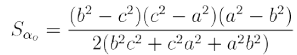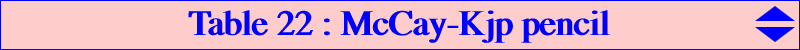The locus of M whose pedal circle C(M) cuts the nine-point circle (N) under a given angle θ is a circum-cubic which is always a K60+ aka a stelloid (and a K0 without term in xyz). More precisely, (N) and C(M) meet at two points M1, M2 which are the centers of the rectangular circum-hyperbolas passing through M and its isogonal conjugate M* respectively. θ is the (directed) angle of the tangents at M1 to (N) and C(M) respectively. With M2, we find another analogous cubic corresponding to the angle –θ. See below for another point of view by Jean-Pierre Ehrmann. The hessian of any such stelloid is a focal cubic with focus G passing through the isodynamic points X(15), X(16). These cubics form a pencil generated by the McCay cubic (the only pK obtained with θ = 0 and tangent circles) and the Kjp cubic (a nK0 obtained with θ = ± π/2 and orthogonal circles). This pencil contains only one K60++ which is K213, the McCay-Ehrmann cubic obtained when θ is the angle of the lines GX(187), GX(511).The general equation is :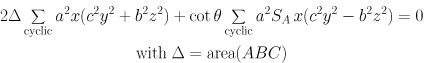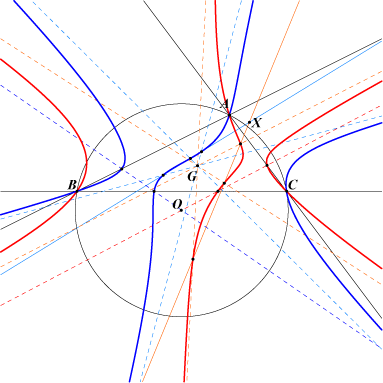The base-points of this pencil are A, B, C and the six anti-points. See Table 77. *** The pencil contains : - two other pKs but they are always imaginary, - two other nK0s (see the opposite figure) which are real when ABC is obtusangle. These two cubics are obtained when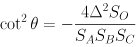The trilinear polars of the roots pass through O and meet the sidelines at three collinear points on the cubics.For any θ, the three real asymptotes concur at the centroid G and are parallel to the altitudes of the Morley triangle rotated of the angle θ. Remark that the tangents at A, B, C are the altitudes of ABC rotated of the angle θ about A, B, C. The polar conics of A, B, C are rectangular hyperbolas with centers the vertices of the second Brocard triangle for any θ. The cubic meets its asymptotes at three collinear points lying on a line L (called the satellite line of the line at infinity) making an angle θ with the parallel at G to the Brocard line OK. For any θ, L contains the point X, homothetic of X(187) under h(G,2/3). X = b^4+c^4-b^2c^2+7a^4-4a^2(b^2+c^2) : : (recall that X(187) in the Schoute center, the inverse of K in the circumcircle and also the intersection of the Brocard and Lemoine axes). Naturally, when the cubic is K213, L is the inflexional tangent at G namely the line GX(187).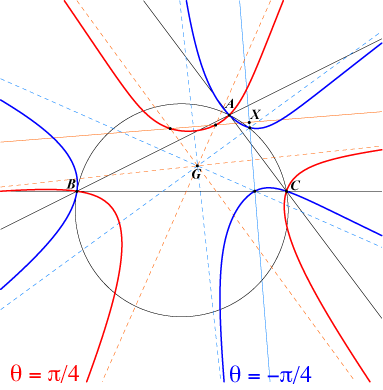For two opposite values of θ, we obtain two conjugate cubics i.e. one of them is the isogonal conjugate of the other one. Following the remark above, the two tangents at a vertex of ABC are symmetric with respect to the altitudes of ABC. It is clear that the McCay cubic and the Kjp cubic are their own conjugates. The figure shows the cubics obtained with θ = ± π/4. The tangents at A, B, C to the two cubics are perpendicular. The asymptote of one of them are the bisectors of the asymptotes of the other. These two cubics meet the circumcircle at A, B, C and six other points which are the vertices of a regular hexagon. Indeed, the isogonal conjugates of the infinite points of one of them are the vertices of an equilateral triangle inscribed in the circumcircle.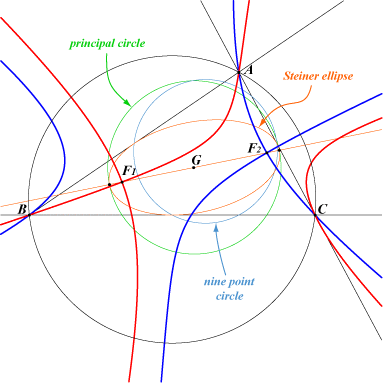The pencil contains two nodal cubics with nodes the real foci F1, F2 of the inscribed Steiner ellipse (there are four other nodal cubics but they are imaginary). The nodal tangents are perpendicular since they are the polar conics of the foci (recall that the polar conic of any point of the plane in a K60+ is a rectangular hyperbola). These two cubics are isogonal conjugates. The corresponding opposite values of $\cot \theta$ are complicated. The common pedal circle of F1 and F2 is the principal circle of the ellipse i.e. the circle with center G and diameter the major axis of the ellipse. Indeed, it is known that the projections of a focus on a tangent to a conic is a point of the principal circle.There are also two axial cubics in this pencil and they are obtained when one of the asymptotes of the stelloid is parallel to one axis of the Steiner ellipse (left hand figure below) Naturally, their hessians are also axial cubics, the curves of the right hand figure below.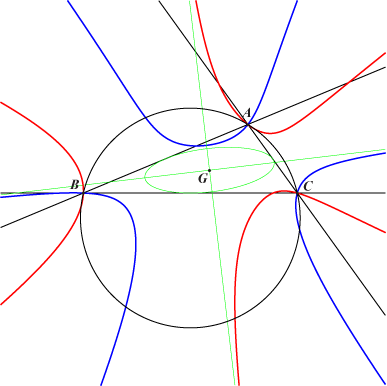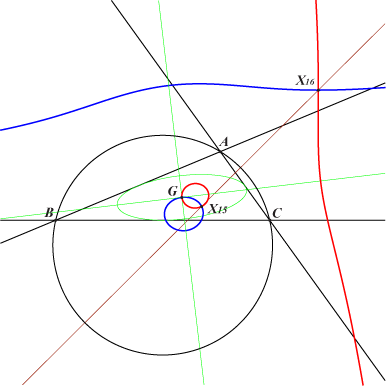Characterization with angles and isogonologic triangles a contribution by Jean-Pierre Ehrmann This generalizes K003 (property 4) and K024 (property 6). See also the yellow cells in Table 7 and Table 8. Let α be a real number. The locus of M such that (BC, AM) + (CA, BM) + (AB, CM) = α (mod. π) is a cubic denoted K(α) with equation : Sα ∑ x (y^2/b^2 + z^2/c^2) + ∑ SA x (y^2/b^2 – z^2/c^2) = 0 where Sα = 2∆ cotα. All these cubics are the members of the pencil generated by the McCay cubic K003 (obtained when α = ± π/2, the second term in the equation) and the Kjp cubic K024 (obtained when α = 0, the first term in the equation) which are the only isogonal cubics of the pencil. More generally, the isogonal transform of K(α) is K(–α). K(α) is a stelloid with three real asymptotes L concurring at G such that (BC, L) + (CA, L) + (AB, L) = α or, equivalently, 3(Lo, L) = α where Lo is a sideline of the Morley triangle. Let now MaMbMc and M* be the circumcevian triangle and the isogonal conjugate of M. K(α) is also the locus of M such that (AM*, MbMc) = α and similarly with the other vertices. This generalizes K024 (property 7). *** Recall that, if T = UVW and T' = U'V'W' are two triangles, then (T, T') is said to be α–isogonologic if and only if the lines L1, L2, L3 passing through U', V', W' respectively and such that (VW, L1) = (WU, L2) = (UV, L3) = α are concurrent. This denomination is due to Duran-Loriga in a paper published in 1902, AFAS pp.157-165. Note that this definition is generally not symmetric unless α is 0 or ± π/2 corresponding to the parallelogic and orthologic special cases. More precisely, if (T, T') is α–isogonologic then (T', T) is (–α)–isogonologic and conversely. Let refM and symM be the triangles formed by the reflections of M in the sidelines and the vertices of ABC respectively. K(α) is then the locus of M such that (T, T') is α–isogonologic in at least six configurations, namely : • T = ABC and T' = MaMbMc.This generalizes K003 (property 13) and K024 (property 8). • T and T' are the pedal triangles of M and M* respectively. This generalizes K003 (property 12) and K024 (property 10). • T and T' are the pedal and antipedal triangles of M respectively. This generalizes K003 (property 17) and K024 (property 12). • T and T' are refM and the antipedal triangle of M respectively. This generalizes K003 (property 23) and K024 (property 14). • T and T' are refM and the circumcevian triangle of M respectively. This generalizes K003 (property 24) and K024 (property 16). • T and T' are symM and the circumcevian triangle of M respectively. This generalizes K003 (property 25) and K024 (property 16). *** Let A', B', C' be the midpoints of ABC. The central stelloid K213 is the member of the pencil which passes through G corresponding to αo = (BC, AA') + (CA, BB') + (AB, CC') and then we obtain :